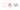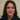# Truthy or Falsy values in Javascript and how to work with them?

JavaScript variables are loosely/dynamically typed and the language doesn’t care how a value is declared or changed. A truthy or falsy value is a value that translates to ture/false when evaluated in a Boolean{.glossaryLink} context. Examples of falsy values in JavaScript (which translate to false and thus bypass the `if`block):

`if (false)`
`if (null)`
`if (undefined)`
`if (0)`
`if (NaN)`
`if ('')`
`if ("")`
`if (document.all)`

### True or false

These are different types of data we can define as:

`let x;`
`x = 1; // x is a number`
`x = '1'; // x is a string`
`x = ; // x is an array`

When using  `== (loose or abstract equality) for comparison, Javascript converts each side to a string and then does the comparison:`

``` // all true 1 == '1'; 1 == ; '1' == ; But using  === (strict equality)  Javascript considers type and all will evaluate to false: // all false 1 === '1'; 1 === ; '1' === ; Internally, JavaScript sets a value to one of six primitive data types: Undefined (a variable with no defined value) Null (a single null value) Boolean (true or false) Number (this includes Infinity and NaN – not a number!) String (textual data) Symbol (a unique and immutable primitive new to ES6/2015) Everything else is an Object — including arrays. How evaluation works As well as a type, each value also has an inherent boolean value, generally known as either truthy or falsy.  So the following values are always falsy: false  (zero) ” or "" (empty string) null undefined NaN (e.g. the result of 1/0) And everything else including these are truthy: ‘0’ (a string containing a single zero, it is not zero) ‘false’ (a string containing the text “false”) [] (this is an empty array) {} (an empty object) function(){} (this is an “empty” function) It means we cannot use a single value for evaluation in statements as it is always true: if (value) { // value is truthy } else { // value is falsy // it could be false, 0, '', null, undefined or NaN } The rules to follow Remember these rules while evaluating truthy and falsy: false, zero and empty strings are all equivalent. null and undefined are equivalent to themselves and each other but nothing else. NaN is not equivalent to anything – including another NaN! Infinity is truthy – but cannot be compared to true or false! An empty array is truthy – yet comparing with true is false and comparing withfalse is true?! These are some examples: // all true false == 0; 0 == ''; null == undefined; [] == false; !! == true; // all false false == null; NaN == NaN; Infinity == true; [] == true;  == true; Use === strict equality and avoid type conversion issues // instead of if (x == y) // ... // runs if x and y are both truthy or both falsy // e.g. x = null and y = undefined // use if (x === y) // ... // runs if x and y are identical... // except when both are NaN Stop direct comparisons It is rare to compare two false or true values: // instead of if (x == false) // ... // runs if x is false, 0, '', or [] // use if (!x) // ... // runs if x is false, 0, '', NaN, null or undefined Convert to real boolean value when necessary Double-negative !! always generates false by false, , "", null, undefinedand NaN. So in this case do as follows: // instead of x or y on the side of comparison if (x === y) // ... // runs if x and y are identical... // except when both are NaN // use double-negative to make sure tey generate what you expect if (!!x === !!y) // ... // runs if x and y are identical... // including when either or both are NaN Always be careful around truthy and falsy values. For example an empty string or a NAN could make the debugging very hard and painful. ```
`© 2020This site is created and maintined by Azadeh Faramarzi , A passionate developer, sport and code lover who loves to share and write when she has some time. `